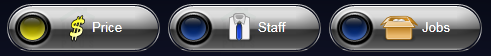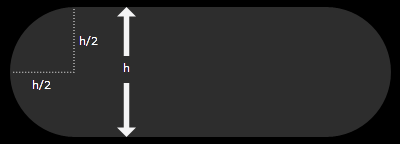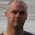## Monday, June 14, 2010

### Introduction

I created a design for a radio button that looks like this:I'm going to write a future post on how to do this button, but I want to refine it a bit first. In this post I’m going to describe the approach I used for the rounded end-caps in the hopes that someone will help me perfect it.

I have the following requirements for this radio button style:
• It must be re-sizable
• The rounded ends must stay perfect half circles in proportion to the height of the button
• It must behave correctly in Blend
• Optional Extra: It would be nice to not have to manually edit XAML in Blend

I have the first three ticked off but the best approach so far still requires editing the XAML by hand. Here is a simple graphic to illustrate the end result when applied to a Rectangle element. The RadiusX and RadiusY are set to half the height of the rectangle:Having the RadiusX and RadiusY calculated automatically is crucial to this design since I want it to be fully scalable (within reason).

I’ve been through several different approaches trying to get the full 4 marks, but 3 is the best I can manage. If you can spot any improvements or alternative approaches I would welcome suggestions.

### Attached Property: 3 out of 4

The winning approach so far is to use an Attached Property that calculates the radii when the rectangle changes size. I made the attached property a little more generic by adding another attached property for specifying a ratio; 0.5 (the default) would give the result above. I also made it work off the lesser of the height and width values to cater for rectangles that are longer than they are wide. Here is the code for the attached property:

`   1: using System;`
`   2: using System.Windows;`
`   3: using System.Windows.Shapes;`
`   4:  `
`   5: /// <summary>`
`   6: /// Contains attached properties for specifiying rounded ends on a Rectangle`
`   7: /// </summary>`
`   8: public static class RoundCaps`
`   9: {`
`  10:     /// <summary>`
`  11:     /// Attached Dependency Property for the IsRounded property`
`  12:     /// </summary>`
`  13:     public static readonly DependencyProperty IsRoundedProperty = DependencyProperty.RegisterAttached(`
`  14:         "IsRounded",`
`  15:         typeof(bool),`
`  16:         typeof(RoundCaps),`
`  17:         new PropertyMetadata(false, IsRoundedChanged));`
`  18:  `
`  19:     /// <summary>`
`  20:     /// Attached Dependency Property for the RoundCapsRatio property`
`  21:     /// </summary>`
`  22:     public static readonly DependencyProperty RoundCapsRatioProperty = DependencyProperty.RegisterAttached(`
`  23:         "RoundCapsRatio",`
`  24:         typeof(double),`
`  25:         typeof(RoundCaps),`
`  26:         new PropertyMetadata(0.5, RoundCapsRatioChanged));`
`  27:  `
`  28:     /// <summary>`
`  29:     /// Gets a value indicating if the Rectangle is rounded.`
`  30:     /// </summary>`
`  31:     /// <param name="d">The rectangle.</param>`
`  32:     /// <returns>true if the rectangle is rounded, else false</returns>`
`  33:     public static bool GetIsRounded(DependencyObject rectangle)`
`  34:     {`
`  35:         return (bool)rectangle.GetValue(IsRoundedProperty);`
`  36:     }`
`  37:  `
`  38:     /// <summary>`
`  39:     /// Sets a value indicating if the Rectangle is rounded.`
`  40:     /// </summary>`
`  41:     /// <param name="rectangle">The rectangle.</param>`
`  42:     /// <param name="value">true if the rectangle is rounded, else false.</param>`
`  43:     public static void SetIsRounded(DependencyObject rectangle, object value)`
`  44:     {`
`  45:         rectangle.SetValue(IsRoundedProperty, value);`
`  46:     }`
`  47:  `
`  48:     /// <summary>`
`  49:     /// Gets the roundedness ratio of the rectangle.`
`  50:     /// </summary>`
`  51:     /// <param name="rectangle">The ratio, relative to the lesser value of the rectangle's height and width.</param>`
`  52:     /// <returns>The ratio currently applied when calculating the roundedness</returns>`
`  53:     public static double GetRoundCapsRatio(DependencyObject rectangle)`
`  54:     {`
`  55:         return (double)rectangle.GetValue(RoundCapsRatioProperty);`
`  56:     }`
`  57:  `
`  58:     /// <summary>`
`  59:     /// Sets the roundedness ratio of the rectangle.`
`  60:     /// </summary>`
`  61:     /// <param name="rectangle">The ratio, relative to the lesser value of the rectangle's height and width.</param>`
`  62:     /// <returns>The ratio to be applied when calculating the roundedness</returns>`
`  63:     public static void SetRoundCapsRatio(DependencyObject d, object value)`
`  64:     {`
`  65:         d.SetValue(RoundCapsRatioProperty, value);`
`  66:     }`
`  67:  `
`  68:     private static void IsRoundedChanged(DependencyObject d, DependencyPropertyChangedEventArgs e)`
`  69:     {`
`  70:         Rectangle rect = d as Rectangle;`
`  71:         if (null == d)`
`  72:             return;`
`  73:  `
`  74:         bool isRounded = (bool)e.NewValue == true;`
`  75:         if (isRounded)`
`  76:         {`
`  77:             rect.SizeChanged += RectSizeChanged;`
`  78:             UpdateRectangle(rect);`
`  79:         }`
`  80:         else`
`  81:         {`
`  82:             rect.SizeChanged -= RectSizeChanged;`
`  83:         }`
`  84:     }`
`  85:  `
`  86:     private static void RoundCapsRatioChanged(DependencyObject d, DependencyPropertyChangedEventArgs e)`
`  87:     {`
`  88:         Rectangle rect = d as Rectangle;`
`  89:         if (null == rect)`
`  90:         {`
`  91:             return;`
`  92:         }`
`  93:  `
`  94:         UpdateRectangle(rect);`
`  95:     }`
`  96:  `
`  97:     private static void RectSizeChanged(object sender, SizeChangedEventArgs e)`
`  98:     {`
`  99:         Rectangle rect = sender as Rectangle;`
` 100:         if (null == rect)`
` 101:         {`
` 102:             return;`
` 103:         }`
` 104:  `
` 105:         UpdateRectangle(rect);`
` 106:     }`
` 107:  `
` 108:     private static void UpdateRectangle(Rectangle rect)`
` 109:     {`
` 110:         double ratio = (double)rect.GetValue(RoundCapsRatioProperty);`
` 111:  `
` 112:         rect.RadiusX = Math.Min(rect.ActualHeight, rect.ActualWidth) * ratio;`
` 113:         rect.RadiusY = rect.RadiusX;`
` 114:     }`
` 115: }`

This is how the XAML looks (without the RoundedRatio property which defaults to 0.5 anyway):

<Rectangle x:Name="rectangle" local:RoundCaps.IsRounded="True" Fill="#FF2D2D2D"/>

This approach works nicely at both run time and design time (1 point each) and is fully scalable (1 point). Given the awkwardness of the other approaches I’ve tried, this is the winning solution so far.

Here are the two other approaches I’ve tried that didn’t fare so well.

### Value Converter: 1 Out Of 4

One approach was to bind the RadiusX and RadiusY properties of the rectangle to itself and use an IValueConverter to calculate the values based off the height and width of the rectangle. In case you’re interested, here is the code for the value converter:

`   1: public class RatioConverter : IValueConverter`
`   2: {`
`   3:  `
`   4:     public object Convert(object value, Type targetType, object parameter, System.Globalization.CultureInfo culture)`
`   5:     {`
`   6:         FrameworkElement element = (FrameworkElement)value;`
`   7:         double height = element.ActualHeight;`
`   8:         double number = height;`
`   9:         double ratio = double.Parse((string)parameter);`
`  10:         return number * ratio;`
`  11:     }`
`  12:  `
`  13:     public object ConvertBack(object value, Type targetType, object parameter, System.Globalization.CultureInfo culture)`
`  14:     {`
`  15:         throw new NotSupportedException();`
`  16:     }`
`  17: }`

The converter doesn’t work at all at run time because it evaluates during the Measure and Arrange cycle for the visual tree, which means that ActualHeight and ActualWidth haven’t actually been calculated and are still 0. I will give it half a point because if I explicitly set the height rather than use “Auto” it does work; but having to explicitly set the height is not good if I’m using this approach inside a resizable Button’s control template.

I will also give it 0.5 for working in Blend – almost. Again, if I explicitly set the height it works in Blend. Blend does somehow manage to apply it correctly for Auto height but only when I build the project (no extra points).

I tried a couple of variations around this, such as binding against the width and height of a sibling element (or cousin) but it started getting a little bit ridiculous and the code-smell was pretty terrible.

### Attached Behavior: The Wrong 3 Out Of 4

Another thing I tried was writing an attached behavior for a Rectangle that would automatically set the corner radius values based on the dimensions of the rectangle. That works fine for a running application, but behaviors don't execute in Blend, so the design-time experience is ruined since the corners remain square.

This is the code for the behavior:

`   1: public class RoundCapsBehavior : Behavior<Rectangle>`
`   2: {`
`   3:     public static readonly DependencyProperty RoundedRatioProperty = DependencyProperty.Register(`
`   4:         "RoundedRatio", typeof(double), typeof(RoundCapsBehavior), new PropertyMetadata(0.5, RoundedRatioChanged));`
`   5:  `
`   6:     public double RoundedRatio`
`   7:     {`
`   8:         get { return (double)this.GetValue(RoundedRatioProperty); }`
`   9:         set { this.SetValue(RoundedRatioProperty, value); }`
`  10:     }`
`  11:  `
`  12:     protected override void OnAttached()`
`  13:     {`
`  14:         base.OnAttached();`
`  15:         this.AssociatedObject.SizeChanged += AssociatedObjectSizeChanged;`
`  16:     }`
`  17:  `
`  18:     protected override void OnDetaching()`
`  19:     {`
`  20:         base.OnDetaching();`
`  21:         this.AssociatedObject.SizeChanged -= AssociatedObjectSizeChanged;`
`  22:     }`
`  23:  `
`  24:     void AssociatedObjectSizeChanged(object sender, SizeChangedEventArgs e)`
`  25:     {`
`  26:         this.UpdateRadiusXY();`
`  27:     }`
`  28:  `
`  29:     private void UpdateRadiusXY()`
`  30:     {`
`  31:         this.AssociatedObject.RadiusX =`
`  32:             Math.Min(this.AssociatedObject.ActualHeight, this.AssociatedObject.ActualWidth)`
`  33:             * this.RoundedRatio;`
`  34:         this.AssociatedObject.RadiusY = this.AssociatedObject.RadiusX;`
`  35:     }`
`  36:  `
`  37:     private static void RoundedRatioChanged(DependencyObject d, DependencyPropertyChangedEventArgs e)`
`  38:     {`
`  39:         RoundCapsBehavior behavior = d as RoundCapsBehavior;`
`  40:         if (null == behavior)`
`  41:             return;`
`  42:         behavior.UpdateRadiusXY();`
`  43:     }`
`  44: }`

It’s pretty simple – it just attaches a handler to the SizeChanged event and recalculates the corners when the event fires. It uses a property on the behavior that you can change the ratio with; the default 0.5 so the radius values will be half the height or width (whichever is larger).

It gets 1 point for working perfectly at run time, and 1 point for being scalable. It also gets the bonus point for not requiring any direct editing of the XAML, which gives it 3 out of 4, but one of those 3 was optional and it missed the third required point.

This one is probably the most frustrating because it feels like it should work. Behaviors, after all, were created as a convenience for the design surface – attached properties can do everything a behavior can – but the OnAttached and OnDetached methods are not called when the behaviors are used inside Blend.

### Summary

So the Attached Dependency Property is currently winning the race, and unless someone comes up with a better solution, it will be the method-of-choice for the radio button style at the top. I just wish that Blend exposed a visual mechanism for adding custom attached properties to elements. Attached Behaviors were meant to give us that, but the OnAttached and OnDetached don’t get called so the behavior is not applied in the design surface.

1.Phil - I've not looked closely at the XAML, but could something like this help?:

http://chriscavanagh.wordpress.com/2008/10/03/wpf-easy-rounded-corners-for-anything/

It uses another element "behind" your real content as an opacity mask (so your content gets clipped to the shape of the mask). I'll kick the idea around a bit and post again if it works...

2.Good idea Chris, but the drawback of using an opacity mask is that the stroke of the rectangle gets clipped too.

I had a look at the link you sent, but that seems to require explicit setting of values and is solving a different problem.

3.As soon as this page loaded, I saw the buttons, and I said, wow those look nice.

4.great work, I was so impressed - I wanted to find out about hiring you.... but no contact details ;(

5.Hi Alex,

just put a dot between my first name and last and put gmail.com on the end of it.